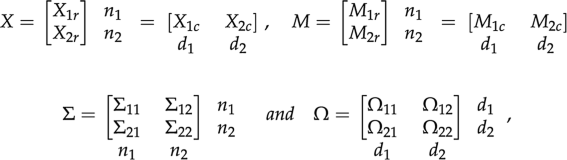# Correction to: Multivariate Gaussian and Student-t process regression for multi-output prediction

The Original Article was published on 31 December 2019

## Correction to: Neural Computing and Applications https://doi.org/10.1007/s00521-019-04687-8

Unfortunately, Theorem 3 was published with incorrect equations in the online publication of the article.

The correct theorem should read as follows:

### Theorem 3

(Marginalization and conditional distribution) Let$$X\sim{\mathcal{M}\mathcal{N}}_{n,d} (M,\varSigma ,\Omega )$$and partition$$X,M,\Sigma$$and$$\Omega$$aswhere$$n_{1} ,n_{2} ,d_{1} ,d_{2}$$is the column or row length of the corresponding vector or matrix. Then,

1. 1.

$$X_{1r} \sim{\mathcal{M}\mathcal{N}}_{{n_{1} ,d}} \left( {M_{1r} ,\Sigma _{11} ,\Omega } \right)$$,

$$X_{2r} |X_{1r} \sim{\mathcal{M}\mathcal{N}}_{{n_{2} ,d}} \left( {M_{2r} +\Sigma _{21}\Sigma _{11}^{ - 1} (X_{1r} - M_{1r} ),\Sigma _{22 \cdot 1} ,\Omega } \right);$$
2. 2.

$$X_{1c} \sim{\mathcal{M}\mathcal{N}}_{{n,d_{1} }} \left( {M_{1c} ,\Sigma ,\Omega _{11} } \right)$$,

$$X_{2c} |X_{1c} \sim{\mathcal{M}\mathcal{N}}_{{n,d_{2} }} \left( {M_{2c} + (X_{1c} - M_{1c} )\Omega _{11}^{ - 1}\Omega _{12} ,\Sigma ,\Omega _{22 \cdot 1} } \right), \,$$

where$$\Sigma _{22 \cdot 1}$$and$$\Omega _{22 \cdot 1}$$are the Schur complement  of$$\Sigma _{11}$$and$$\Omega _{11}$$, respectively,

$$\Sigma _{22 \cdot 1} =\Sigma _{22} -\Sigma _{21}\Sigma _{11}^{ - 1}\Sigma _{12} ,\quad\Omega _{22 \cdot 1} =\Omega _{22} -\Omega _{21}\Omega _{11}^{ - 1}\Omega _{12} . \,$$

The original article has been updated accordingly.

## Author information

Authors

### Corresponding author

Correspondence to Zexun Chen.